# Python | Linear Regression using sklearn

## Python | Linear Regression using sklearn

Linear Regression is a machine learning algorithm based on supervised learning. It performs a regression task. Regression models a target prediction value based on independent variables. It is mostly used for finding out the relationship between variables and forecasting. Different regression models differ based on – the kind of relationship between dependent and independent variables, they are considering and the number of independent variables being used.

This article is going to demonstrate how to use the various Python libraries to implement linear regression on a given dataset. We will demonstrate a binary linear model as this will be easier to visualize.

In this demonstration, the model will use Gradient Descent to learn. You can learn about it here.

Step 1: Importing all the required libraries

 `import` `numpy as np` `import` `pandas as pd` `import` `seaborn as sns` `import` `matplotlib.pyplot as plt` `from` `sklearn ``import` `preprocessing, svm` `from` `sklearn.model_selection ``import` `train_test_split` `from` `sklearn.linear_model ``import` `LinearRegression`

 `cd C:\Users\Dev\Desktop\Kaggle\Salinity` ` ` `# Changing the file read location to the location of the dataset` `df ``=` `pd.read_csv(``'bottle.csv'``)` `df_binary ``=` `df[[``'Salnty'``, ``'T_degC'``]]` ` ` `# Taking only the selected two attributes from the dataset` `df_binary.columns ``=` `[``'Sal'``, ``'Temp'``]` ` ` `# Renaming the columns for easier writing of the code` `df_binary.head()` ` ` `# Displaying only the 1st  rows along with the column names`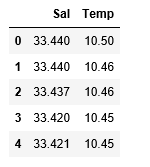Step 3: Exploring the data scatter

 `sns.lmplot(x ``=``"Sal"``, y ``=``"Temp"``, data ``=` `df_binary, order ``=` `2``, ci ``=` `None``)` ` ` `# Plotting the data scatter`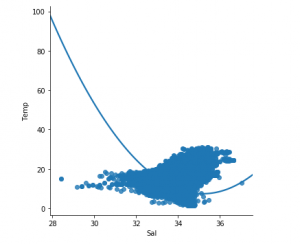Step 4: Data cleaning

 `# Eliminating NaN or missing input numbers` `df_binary.fillna(method ``=``'ffill'``, inplace ``=` `True``)`

Step 5: Training our model

 `X ``=` `np.array(df_binary[``'Sal'``]).reshape(``-``1``, ``1``)` `y ``=` `np.array(df_binary[``'Temp'``]).reshape(``-``1``, ``1``)` ` ` `# Separating the data into independent and dependent variables` `# Converting each dataframe into a numpy array ` `# since each dataframe contains only one column` `df_binary.dropna(inplace ``=` `True``)` ` ` `# Dropping any rows with Nan values` `X_train, X_test, y_train, y_test ``=` `train_test_split(X, y, test_size ``=` `0.25``)` ` ` `# Splitting the data into training and testing data` `regr ``=` `LinearRegression()` ` ` `regr.fit(X_train, y_train)` `print``(regr.score(X_test, y_test))`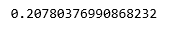Step 6: Exploring our results

 `y_pred ``=` `regr.predict(X_test)` `plt.scatter(X_test, y_test, color ``=``'b'``)` `plt.plot(X_test, y_pred, color ``=``'k'``)` ` ` `plt.show()` `# Data scatter of predicted values`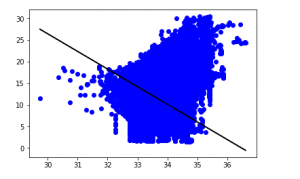The low accuracy score of our model suggests that our regressive model has not fitted very well to the existing data. This suggests that our data is not suitable for linear regression. But sometimes, a dataset may accept a linear regressor if we consider only a part of it. Let us check for that possibility.

Step 7: Working with a smaller dataset

 `df_binary500 ``=` `df_binary[:][:``500``]` ` ` `# Selecting the 1st 500 rows of the data` `sns.lmplot(x ``=``"Sal"``, y ``=``"Temp"``, data ``=` `df_binary500,` `                               ``order ``=` `2``, ci ``=` `None``)`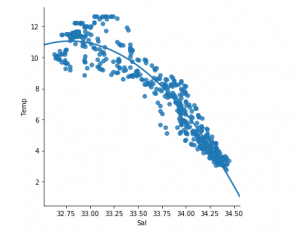We can already see that the first 500 rows follow a linear model. Continuing with the same steps as before.

 `df_binary500.fillna(method ``=``'ffill'``, inplace ``=` `True``)` ` ` `X ``=` `np.array(df_binary500[``'Sal'``]).reshape(``-``1``, ``1``)` `y ``=` `np.array(df_binary500[``'Temp'``]).reshape(``-``1``, ``1``)` ` ` `df_binary500.dropna(inplace ``=` `True``)` `X_train, X_test, y_train, y_test ``=` `train_test_split(X, y, test_size ``=` `0.25``)` ` ` `regr ``=` `LinearRegression()` `regr.fit(X_train, y_train)` `print``(regr.score(X_test, y_test))`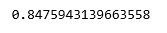`y_pred ``=` `regr.predict(X_test)` `plt.scatter(X_test, y_test, color ``=``'b'``)` `plt.plot(X_test, y_pred, color ``=``'k'``)` ` ` `plt.show()`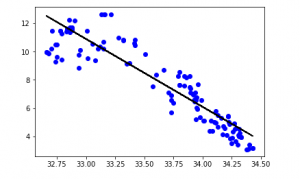Last Updated on March 17, 2022 by admin

## Django ORM – Inserting, Updating & Deleting DataDjango ORM – Inserting, Updating & Deleting Data

Django ORM – Inserting, Updating & Deleting Data Django lets us interact with its database

## Django Project MVT StructureDjango Project MVT Structure

Django Project MVT Structure Django is based on MVT (Model-View-Template) architecture. MVT is a software design pattern

## os.rename() method – Pythonos.rename() method – Python

Python | os.rename() method OS module in Python provides functions for interacting with the operating system.

## numpy.sqrt() in Pythonnumpy.sqrt() in Python

numpy.sqrt() in Python numpy.sqrt(array[, out]) function is used to determine the positive square-root of an array,

## Get all rows in a Pandas DataFrame containing given substringGet all rows in a Pandas DataFrame containing given substring

Get all rows in a Pandas DataFrame containing given substring Let’s see how to get

## Python program to get all unique combinations of two ListsPython program to get all unique combinations of two Lists

Python program to get all unique combinations of two Lists The combination is a mathematical

## close() and quit() method in Selenium Pythonclose() and quit() method in Selenium Python

How to use close() and quit() method in Selenium Python ? While doing stuff with

## User-defined Exceptions in Python with ExamplesUser-defined Exceptions in Python with Examples

User-defined Exceptions in Python with Examples Python throws errors and exceptions when there is a